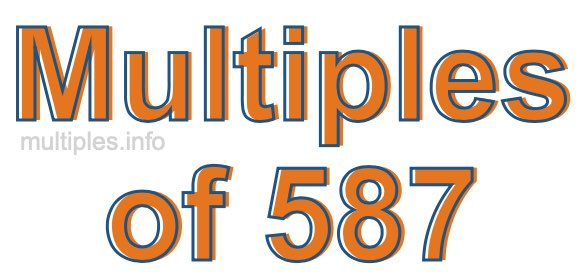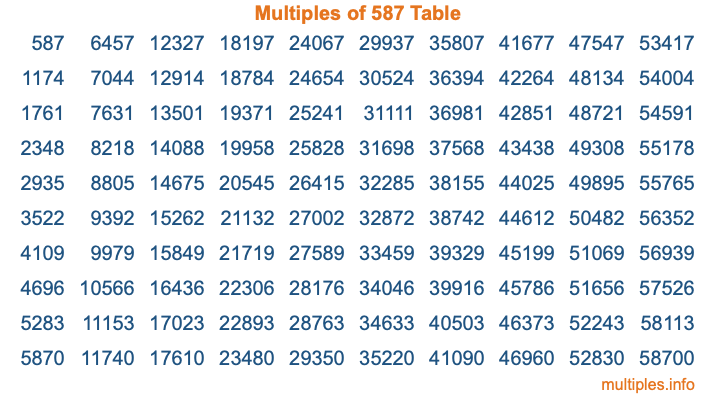Multiples of 587Welcome to the Multiples of 587 page. Here we will first teach you everything you will ever need to know about the multiples of 587, and then give you a study guide summary of everything we taught you to make sure you remember it all. Use this page to look up facts and learn information about the multiples of 587. This page will make you a multiples of five hundred eighty-seven expert!

Definition of Multiples of 587
Multiples of 587 are all the numbers that when divided by 587 equal an integer. Each of the multiples of 587 are called a multiple. A multiple of 587 is created by multiplying 587 by an integer.

Therefore, to create a list of multiples of 587, you start with 1 multiplied by 587, then 2 multiplied by 587, then 3 multiplied by 587, and so on for as long as you want. Thus, the list of the first five multiples of 587 is 587, 1174, 1761, 2348, and 2935. To see a larger list of multiples of 587, see the printable image of Multiples of 587 further down on this page. We also have a category where you can choose any nth multiple of 587.

Multiples of 587 Checker
The Multiples of 587 Checker below checks to see if any number of your choice is a multiple of 587. In other words, it checks to see if there is any number (integer) that when multiplied by 587 will equal your number. To do that, we divide your number by 587. If the the quotient is an integer, then your number is a multiple of 587.

Is  a multiple of 587?

Least Common Multiple of 587 and ...
A Least Common Multiple (LCM) is the lowest multiple that two or more numbers have in common. This is also called the smallest common multiple or lowest common multiple and is useful to know when you are adding our subtracting fractions. Enter one or more numbers below (587 is already entered) to find the LCM.

Check out our LCM Calculator if you need more details about the Least Common Multiple or if you need the LCM for different numbers for adding and subtraction fractions.

nth Multiple of 587
As we stated above, 587 is the first multiple of 587, 1174 is the second multiple of 587, 1761 is the third multiple of 587, and so on. Enter a number below to find the nth multiple of 587.

th multiple of 587

Multiples of 587 vs Factors of 587
587 is a multiple of 587 and a factor of 587, but that is where the similarities end. All postive multiples of 587 are 587 or greater than 587. All positive factors of 587 are 587 or less than 587.

Below is the beginning list of multiples of 587 and the factors of 587 so you can compare:

Multiples of 587: 587, 1174, 1761, 2348, 2935, etc.

Factors of 587: 1, 587

As you can see, the multiples of 587 are all the numbers that you can divide by 587 to get a whole number. The factors of 587, on the other hand, are all the whole numbers that you can multiply by another whole number to get 587.

It's also interesting to note that if a number (x) is a factor of 587, then 587 will also be a multiple of that number (x).

Multiples of 587 vs Divisors of 587
The divisors of 587 are all the integers that 587 can be divided by evenly. Below is a list of the divisors of 587.

Divisors of 587: 1, 587

The interesting thing to note here is that if you take any multiple of 587 and divide it by a divisor of 587, you will see that the quotient is an integer.

Multiples of 587 Table
Below is an image of the first 100 multiples of 587 in a table. The table is in chronological order, column by column. The first column has the first ten multiples of 587, the second column has the next ten multiples of 587, and so on.The Multiples of 587 Table is also referred to as the 587 Times Table or Times Table of 587. You are welcome to print out our table for your studies.

Negative Multiples of 587
Although not often discussed or needed in math, it is worth mentioning that you can make a list of negative multiples of 587 by multiplying 587 by -1, then by -2, then by -3, and so on, to get the following list of negative multiples of 587:

-587, -1174, -1761, -2348, -2935, etc.

Multiples of 587 Summary
Below is a summary of important Multiples of 587 facts that we have discussed on this page. To retain the knowledge on this page, we recommend that you read through the summary and explain to yourself or a study partner why they hold true.

There are an infinite number of multiples of 587.

A multiple of 587 divided by 587 will equal a whole number.

587 divided by a factor of 587 equals a divisor of 587.

The nth multiple of 587 is n times 587.

The largest factor of 587 is equal to the first positive multiple of 587.

587 is a multiple of every factor of 587.

587 is a multiple of 587.

A multiple of 587 divided by a divisor of 587 equals an integer.

587 divided by a divisor of 587 equals a factor of 587.

Any integer times 587 will equal a multiple of 587.

Multiples of a Number
Here you can get the multiples of another number, all with the same attention to detail as we did for multiples of 587 on this page.

Multiples of
Multiples of 588
Did you find our page about multiples of five hundred eighty-seven educational? Do you want more knowledge? Check out the multiples of the next number on our list!